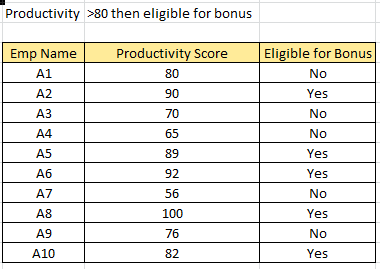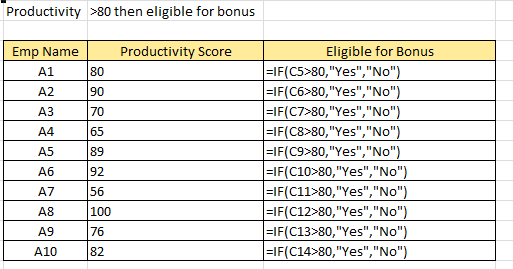## Excel If Function

This function is most of the time used, when you need to determine whether the value is True or False and based on that condition, show some output or execute some action. We will learn how to use this function using few examples.

Below is the standard syntax used by IF function:
=IF(logical_test, value_if_true, value_if_false)

below is the break up and details of the above syntax:

logical_test: here we need to mention the logic that we are going to test. for e.g. if we need to find out if a cell value is greater than 100 (>100), then True else False. We can also test for text value, e.g. if the cell value contains INDIA, then True else False.

value_if_true: if our logical test is True, whatever value we write here, will be the output. e.g. =if(A2>100,”Yes”) here if the value in cell A2 is greater than 100, then the output value will be Yes, else the output value will be False.

value_if_false: if our logical test is False, whatever value we mention here, will show in output. e.g, =if(A2>100,”Yes”,”No”) here if the value in cell A2 is less than 100, then the output value will be No, else the output value will be Yes.

Now we know what exactly the syntax of IF function means. Using this function we can check multiple cell values, based on our logical conditions and use multiple IF conditions, which we call as Nested IF formula. There is limit to use nested IF, which is 64 nested conditions can be tested in single formula.

Lets use one example to test the IF formula. In below example we have 10 employees, for whom we need to find out who all are eligible for Bonus. The bonus eligibility criteria is, if productivity score is greater than 80 then Yes, else no.The formula which we have used in above example, under column Eligible for Bonus is:

=IF(productivity score>80,”Yes”,”No”), while the productivity score is the cell reference, see below image, in our case the productivity score is starting from cell C5.